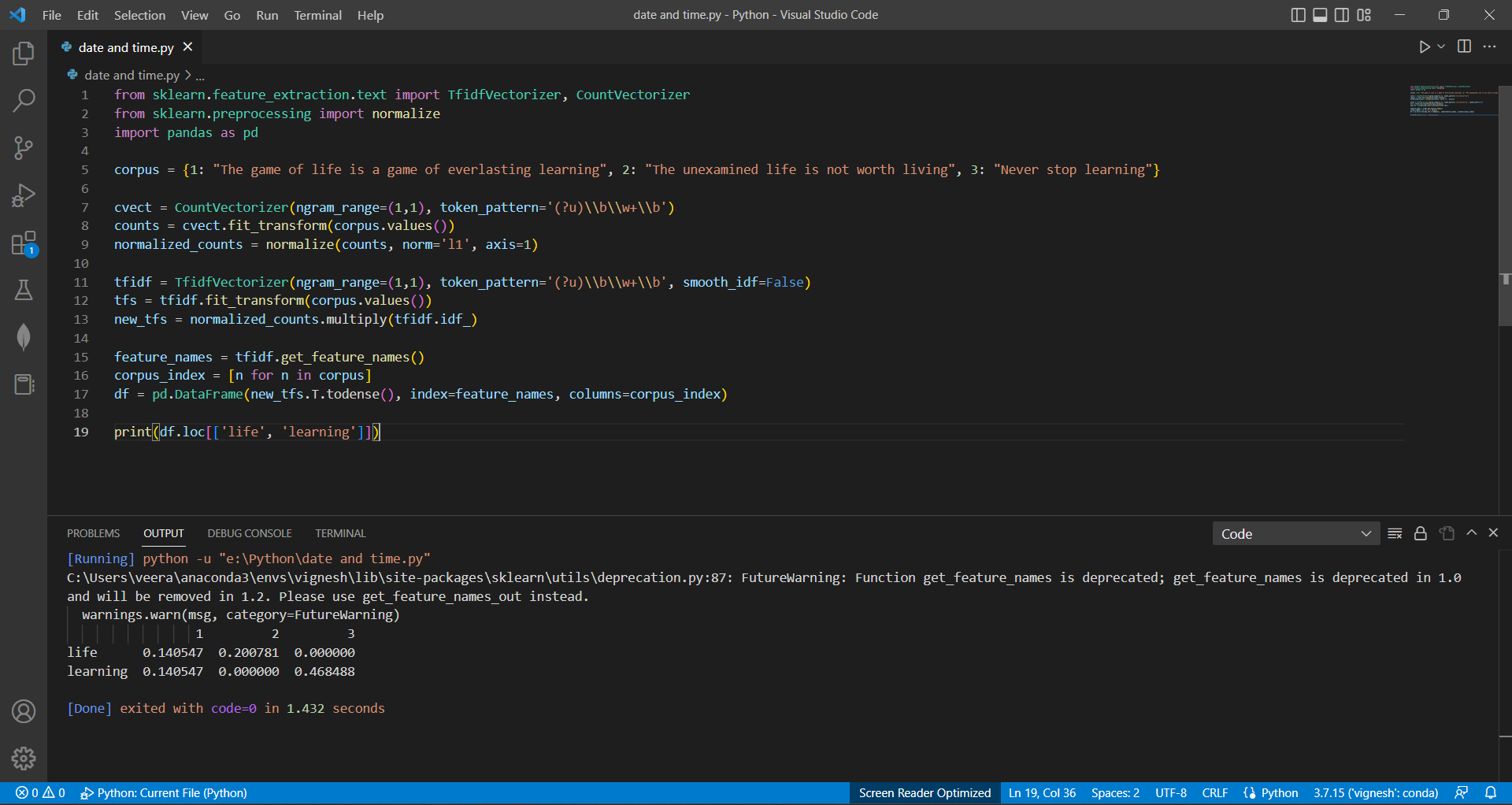# How to Calculate Normalized Tf-Idf Values for a Corpus of Textby vigneshchennai74Updated: Apr 6, 2023Solution Kit

It creates a tf-idf matrix from a corpus of text. Tf-idf (term frequency-inverse document frequency) is a numerical statistic intended to reflect how important a word is to a document in a corpus.

The applications involve working with text data, such as text classification, text clustering, text retrieval, and text summarization. In these applications, the tf-idf values can be used as features for machine learning algorithms or as a representation of the text data for other purposes. Tf-idf is a commonly used technique in text analysis and information retrieval, as it provides a numerical representation of the importance of each word in each document.

• TfidfVectorizer: This class implements the tf-idf (term frequency-inverse document frequency) method for text feature extraction. It is used to convert a collection of raw documents to a matrix of tf-idf values, which can then be used as features for machine learning algorithms.
• CountVectorizer: This class implements a tokenizing and counting method for text feature extraction. It is used to convert a collection of raw documents to a matrix of token counts, which can be used as features for machine learning algorithms.
• normalize: This function is used to normalize a matrix, typically by dividing each row by the sum of its elements

The TfidfVectorizer and CountVectorizer classes and the normalize function are useful in natural language processing (NLP) and text analysis. They are part of the scikit-learn library, a widely used machine-learning library in Python.Preview of the output that you will get on running this code from your IDE

### Code

In this solution we have used TfidfVectorizer .

1. Copy the code using the "Copy" button above, and paste it in a Python file in your IDE.
2. Run the file to get the output

I hope you found this useful. I have added the link to dependent libraries, version information in the following sections.

I found this code snippet by searching for "Check the tf-idf scores of sklearn in python" in kandi. You can try any such use case!

### Environment Tested

I tested this solution in the following versions. Be mindful of changes when working with other versions.

1. The solution is created in Python 3.7.15 version
2. The solution is tested on scikit-learn 1.0.2 version

Using this solution, we are able calculate the TF-IDF values using Scikit learn library in Python with simple steps. This process also facilities an easy to use, hassle free method to create a hands-on working version of code which would help calculate the TF-IDF values using sklearn in Python.

### Dependent Library

scikit-learnby scikit-learn

Python54472Version:1.2.2scikit-learn: machine learning in Python

Support
Quality
Security
Reuse

scikit-learnby scikit-learn

Python54472Version:1.2.2License: Permissive (BSD-3-Clause)

scikit-learn: machine learning in Python
Support
Quality
Security
Reuse

pandasby pandas-dev

Python38552Version:v2.0.2Flexible and powerful data analysis / manipulation library for Python, providing labeled data structures similar to R data.frame objects, statistical functions, and much more

Support
Quality
Security
Reuse

pandasby pandas-dev

Python38552Version:v2.0.2License: Permissive (BSD-3-Clause)

Flexible and powerful data analysis / manipulation library for Python, providing labeled data structures similar to R data.frame objects, statistical functions, and much more
Support
Quality
Security